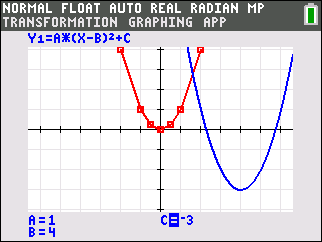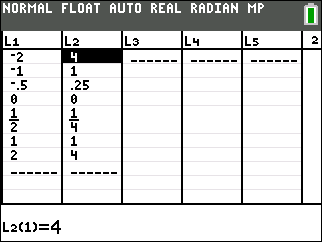# Activities

••• ##### Subject Area

• Math: Algebra I: Quadratic Functions

• ##### Author9-12

60 Minutes

• ##### Device
• TI-84 Plus
• TI-84 Plus Silver Edition
•TI-84 Plus C Silver Edition
•TI-84 Plus CE
• ##### Software

TI Connect™ CE

• ##### Report an Issue#### Activity Overview

Students graph quadratic functions and study how the constants in the equations compare to the coordinates of the vertices and the axes of symmetry in the graphs. The first part of the activity focuses on the vertex form, while the second part focuses on the standard form. Both activities include opportunities for students to pair up and play a graphing game to test how well they really understand the equations of quadratic functions.

#### Key Steps

•Students will graph a quadratic function y = ax2 + bx + c and display a table for integer values of the variable.

•Students will determine the vertex, zeros, and the equation of the axis of symmetry of the graph y = x2 + k and deduce the vertex, the zeros, and the equation of the axis of symmetry of the graph of y = a(x – h)2 + k.

•Students will graph the equation y = a(x – h)2 for various values of a and describe its relationship to the graph of y = (x – h)2.